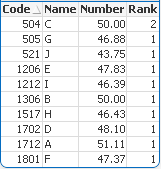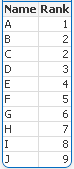# New to QlikView

Discussion board where members can get started with QlikView.

Highlighted
Contributor III

## Rank function for repeated data

Hi All

I have a problem in Rank function.

my data i like this :

 code Number Name 1712 51.11 A 1306 50.00 B 504 50.00 C 1702 48.10 D 1206 47.83 E 1801 47.37 F 505 46.88 G 1517 46.43 H 1212 46.39 I 521 43.75 J

I want to show top 10 Name in text object in sort I use this

=Aggr(if(   rank(sum(Number))=1  ,Name),Code)

It is work for non repeated data but for repeated data its not work. what should I do to show data.

qvw file is attached .

1 Solution

Accepted Solutions
Contributor III

## Re: Rank function for repeated data

I found It, the correct answer is that use :

=Aggr(if(  rank(sum(Number),4,0)=2  ,Name),Code)

9 RepliesMVP

## Re: Rank function for repeated data

Is This you are expectingBefore develop something, think If placed (The Right information | To the right people | At the Right time | In the Right place | With the Right context)
Contributor III

## Re: Rank function for repeated data

No, In my qvw file i explain what I expectMVP

## Re: Rank function for repeated data

I don't have license. For this what are you expectingg

Before develop something, think If placed (The Right information | To the right people | At the Right time | In the Right place | With the Right context)
Contributor III

## Re: Rank function for repeated data

This is what show and what I need.

I use rank for 1 to 10 in text object but in 2 and 3 its not show.MVP

## Re: Rank function for repeated data

Might be thisRowNo(total) - rangesum(above(total if(num(rank(total Number,1))=above(num(rank(total Number,1))),1,0),

0,RowNo(total)))

Before develop something, think If placed (The Right information | To the right people | At the Right time | In the Right place | With the Right context)
Contributor III

## Re: Rank function for repeated data

hmm, not actually.

I want to Show order of rank name in  text object not in chart.

for example I want to show the 2nd number name in thext object. means show B or C.

When I use this  [  Aggr(if(   rank(sum(Number))=2  ,Name),Code) ]    in text onject is show -.MVP & Luminary

## Re: Rank function for repeated data

Maybe this is helpful: Continuous Ranking (no missing Rank)

- Marcus

Contributor III

## Re: Rank function for repeated data

I found It, the correct answer is that use :

=Aggr(if(  rank(sum(Number),4,0)=2  ,Name),Code)MVP

## Re: Rank function for repeated data

Just to add another set of possibility...

=FirstSortedValue(Name, -Aggr(Sum(Number)*100000 - Ord(Name), Code))

=FirstSortedValue(Name, -Aggr(Sum(Number)*100000 - Ord(Name), Code), 2)

=FirstSortedValue(Name, -Aggr(Sum(Number)*100000 - Ord(Name), Code), 3)

=FirstSortedValue(Name, -Aggr(Sum(Number)*100000 - Ord(Name), Code), 4)

=FirstSortedValue(Name, -Aggr(Sum(Number)*100000 - Ord(Name), Code), 5)

=FirstSortedValue(Name, -Aggr(Sum(Number)*100000 - Ord(Name), Code), 6)